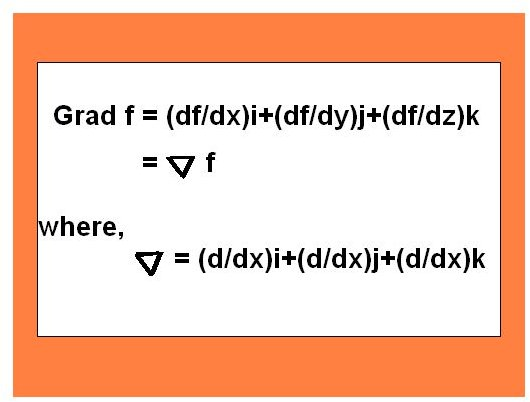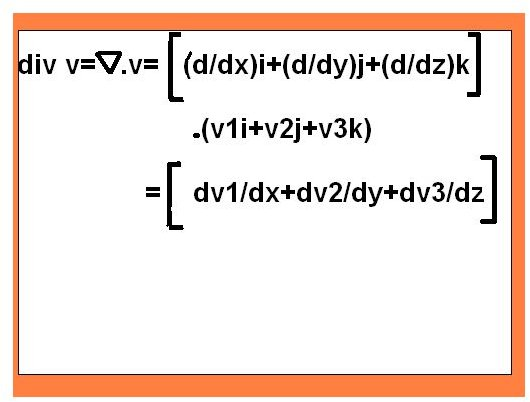# Elements of Vector Analysis – Gradient, Divergence, and Curl

Vector calculus owes much of its importance in engineering and physics to the gradient, divergence, and curl. These terms are used for simplifying the complicated vectors. Gradient, divergence, and curl are basic in connection with fields.

## Gradient of Scalar field

We all know that a scalar field can be solved more easily as compared to vector field. Therefore, it is better to convert a vector field to a scalar field. Not all vector fields can be changed to a scalar field; however, many of them can be changed.

The relation between the two types of fields is accomplished by the term gradient. Hence, gradient of a vector field has a great importance for solving them.

The gradient ‘grad f’ of a given scalar function f(x, y, z) is the vector function expressed as

Grad f = (df/dx) i + (df/dy) j + (df/dz) k

We can also show the above formula in terms of ‘nabla’ and the new form of this formula is expressed by the image below.Let us discuss an example.

If a function is a f(x, y, z) = 2x+ yz – 3y2, then grad f= f= 2i+ (z-6y)j+ yk. Actually, the term df/dx is the partial differentiation with respect to variable x.

• We can change the vector field into a scalar field only if the given vector is differential. The given vector must be differential to apply the gradient phenomenon.
• The gradient of any scalar field shows its rate and direction of change in space.

Having discussed the gradient, we turn next to the divergence.

## Divergence of Vector Field

Let v(x, y, z) be a differentiable vector function, where x, y, and z are Cartesian coordinates, and let v1, v2, v3 be the components of v. Then the function of determining divergence will be expressed as

Div v = d v1/dx + d v2/dy + d v3/dz

This is the formula of determining divergence of a vector. But the condition is same; the given vector must be differentiable.

It can also be expressed in terms of nabla, which is expressed in the image below.Properties of Divergence

• Divergence has an important physical meaning. The values of a function that indicate a physical or geometrical property must be independent of the particular choice of coordinates; that is, those value must be invariant with respect to coordinate transformation. Divergence has the same type of property; it only depends on the points in space and the function (v) but not on the particular choice of coordinates.
• The concept of divergence is used in fluid mechanics.
• This important term is used for expressing conservation of mass or continuity equation.
• If a flow is steady and density of the flow is constant , then the equation of continuity is expressed as

div v = 0

This relation is also known as a condition of incompressibility.

## Curl of Vector Field

Suppose x, y, z are right-handed Cartesian coordinates and v is a function in terms of these variables and is expressed as

V(x, y, z) = v1i + v2j + v3k

The function v is a differentiable vector. Then the curl of vector v will be expressed as

Curl v = [d v3/dy – d v2/dz] i +[d v1/dz – d v3/dx] j + [d v2/dx – d v1/dy] k

This expression is called the curl of a vector field and the curl of a vector field can be solved using this expression.

Properties of curl of vector field

• In the case of a rotation of a rigid body, the curl of the velocity field has the direction of the axis of rotation, and its magnitude equals twice the angular speed of the rotation.
• The curl of a gradient function is ‘0’. Hence, if a vector function is the gradient of a scalar function, its curl is the zero vector.
• The curl function is used for representing the characteristics of the rotation in a field.
• The divergence of a curl function is a zero vector.
• The length and direction of a curl function does not depend on the choice of coordinates system I space.

## Conclusion

It's easy to understand gradient divergence and curl theoretically. However, you have to practice these formulas very well so as they can be used in various mathematical problems. They are large formulas but you can be familiar with them through a good practice.

## Reference

Kreyszig- Advanced Engineering Mathematics